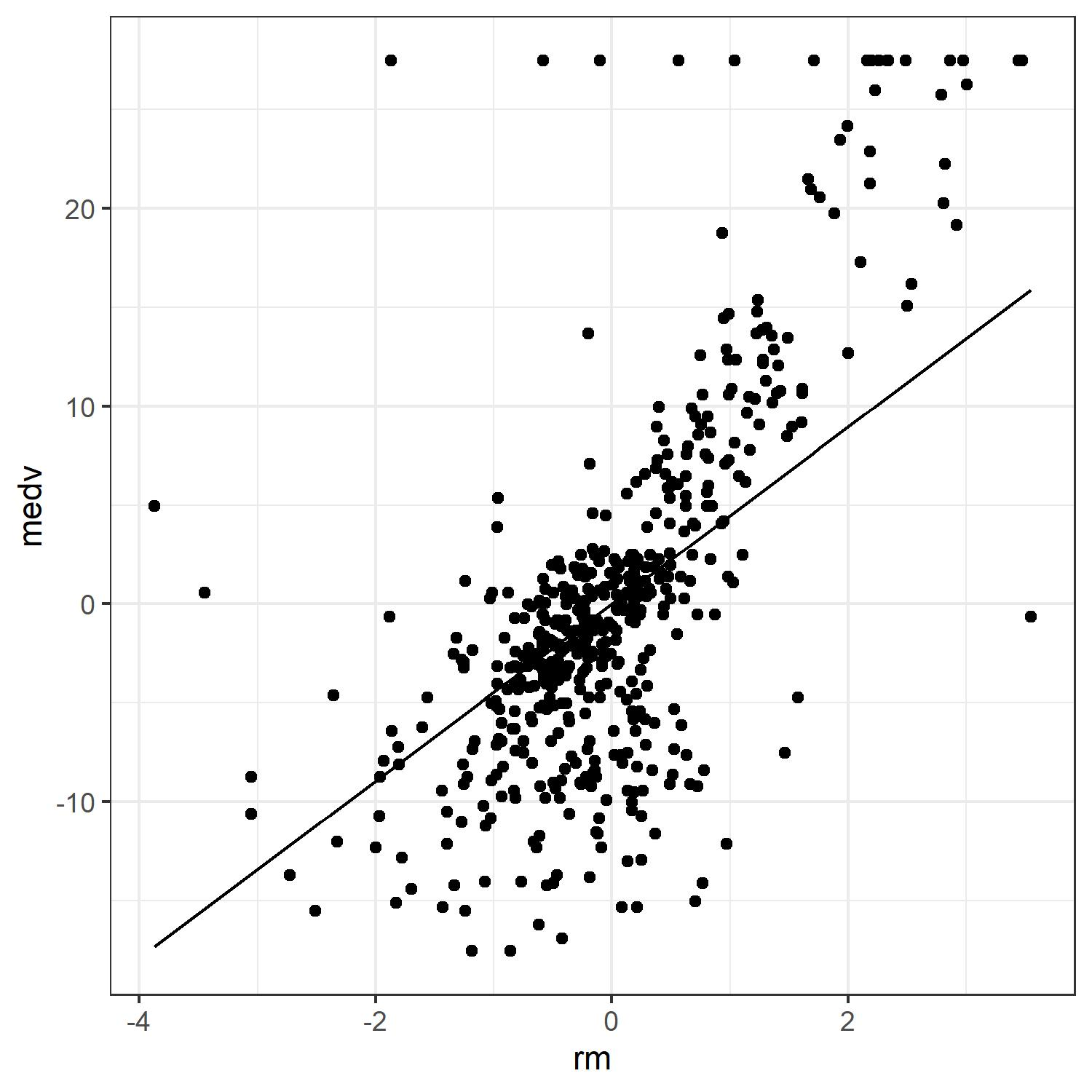# robustlm: Robust Variable Selection with Exponential Squared Loss

The goal of the robustlm package is to carry out robust variable selection through exponential squared loss (Wang et al. 2013). Specifically, it solves the following optimization problem:

$\arg\min_{\beta} \sum_{i=1}^n(1-\exp\{-(y_i-x_i^T\beta)^2/\gamma_n\})+n\sum_{i=1}^d \lambda_{n j}|\beta_{j}|,$ where penalty function is the well-known adaptive LASSO (Zou 2006). $$y_i$$s are responses and $$x_i$$ are predictors. $$\gamma_n$$ is the tuning parameter controlling the degree of robustness and efficiency of the regression estimators. Block coordinate gradient descent algorithm (Tseng and Yun 2009) is used to efficiently solve the optimization problem. The tuning parameter $$\gamma_n$$ and regularization parameter $$\lambda_{nj}$$ are chosen adaptively by default, while they can be supplied by the user.

## Quick Start

This is a basic example which shows you how to use this package. First, we generate data which contain influential points in the response. Let covariate $$x_i$$ follows a multivariate normal distribution $$N(0, \Sigma)$$ where $$\Sigma_{ij}=0.5^{|i-j|}$$, and the error term $$\epsilon$$ follows a mixture normal distribution $$0.8N(0,1)+0.2N(10,6^2)$$. The response is generated by $$Y=X^T\beta+\epsilon$$, where $$\beta=(1, 1.5, 2, 1, 0, 0, 0, 0)^T$$.

set.seed(1)
library(MASS)
N <- 1000
p <- 8
rho <- 0.5
beta_true <- c(1, 1.5, 2, 1, 0, 0, 0, 0)
H <- abs(outer(1:p, 1:p, "-"))
V <- rho^H
X <- mvrnorm(N, rep(0, p), V)

# generate error term from a mixture normal distribution
components <- sample(1:2, prob=c(0.8, 0.2), size=N, replace=TRUE)
mus <- c(0, 10)
sds <- c(1, 6)
err <- rnorm(n=N,mean=mus[components],sd=sds[components])

Y <- X %*% beta_true + err

Regression diagnostics for simple linear regression provide some intuition about the data.

plot(lm(Y ~ X))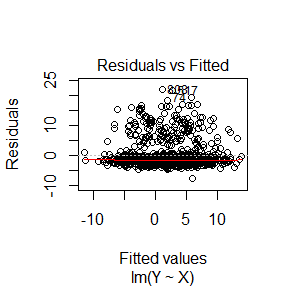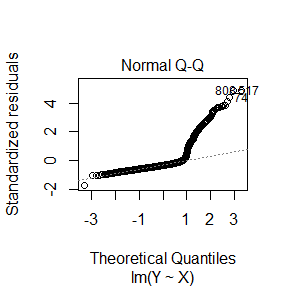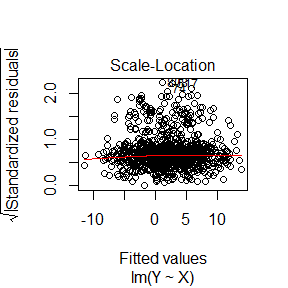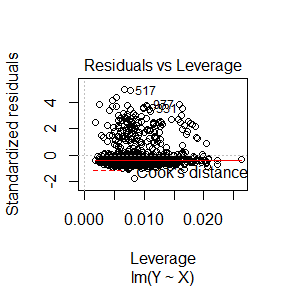The Q-Q plot suggests the normal assumption is not valid. Thus, a robust variable selection procedure is needed. We apply robustlm function to select important variables:

library(robustlm)
robustlm1 <- robustlm(X, Y)
robustlm1
#> $beta #>  0.9411568 1.5839011 2.0716890 0.9489619 0.0000000 0.0000000 0.0000000 #>  0.0000000 #> #>$alpha
#>  0
#>
#> $gamma #>  8.3 #> #>$weight
#>    87.140346    7.033846    4.340160    3.343782    6.833033  703.863830
#>   193.860493  858.412613 2183.876884
#>
#> $loss #>  250.3821 The estimated regression coefficients $$(0.94, 1.58, 2.07, 0.95, 0.00, 0.00, 0.00, 0.00)$$ are close to the true values$$(1, 1.5, 2, 1, 0, 0, 0, 0)$$. There is no mistaken selection or discard. robustlm package also provides generic coef function to extract estimated coefficients and predict function to make a prediction: coef(robustlm1) #>$alpha
#>  0
#>
#> $beta #>  0.9411568 1.5839011 2.0716890 0.9489619 0.0000000 0.0000000 0.0000000 #>  0.0000000 Y_pred <- predict(robustlm1, X) head(Y_pred) #> [,1] #> [1,] 4.3571855 #> [2,] 0.2831909 #> [3,] 3.0404662 #> [4,] -1.1539956 #> [5,] 0.9718605 #> [6,] -0.5732342 ## Boston Housing Price Dataset We apply this package to analysis the Boston Housing Price Dataset, which is available in MASS package. The data contain 14 variables and 506 observations. The response variable is medv (median value of owner-occupied homes in thousand dollars), and the rest are the predictors. Here the predictors are scaled to have zero mean and unit variance. The responses are centerized. data(Boston, package = "MASS") head(Boston) #> crim zn indus chas nox rm age dis rad tax ptratio black lstat #> 1 0.00632 18 2.31 0 0.538 6.575 65.2 4.0900 1 296 15.3 396.90 4.98 #> 2 0.02731 0 7.07 0 0.469 6.421 78.9 4.9671 2 242 17.8 396.90 9.14 #> 3 0.02729 0 7.07 0 0.469 7.185 61.1 4.9671 2 242 17.8 392.83 4.03 #> 4 0.03237 0 2.18 0 0.458 6.998 45.8 6.0622 3 222 18.7 394.63 2.94 #> 5 0.06905 0 2.18 0 0.458 7.147 54.2 6.0622 3 222 18.7 396.90 5.33 #> 6 0.02985 0 2.18 0 0.458 6.430 58.7 6.0622 3 222 18.7 394.12 5.21 #> medv #> 1 24.0 #> 2 21.6 #> 3 34.7 #> 4 33.4 #> 5 36.2 #> 6 28.7 # scaling and centering Boston[, -14] <- scale(Boston[, -14]) Boston[, 14] <- scale(Boston[, 14], scale = FALSE) # diagnostic set.seed(1) x <- as.matrix(Boston[, -14]) y <- Boston[, 14] lm_OLS <- lm(y ~ x - 1) plot(lm_OLS)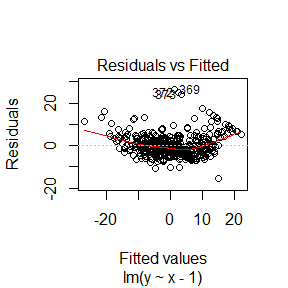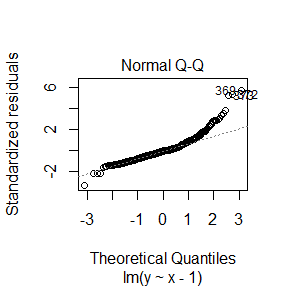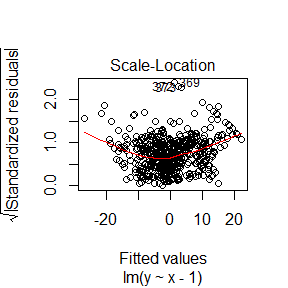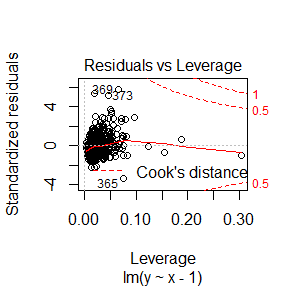The diagnostic plots suggest the residuals may not follow normal distribution, we use robustlm to carry out variable selection with robustness. # robustlm robustlm2 <- robustlm(x, y) coef(robustlm2) #>$alpha
#>  -0.8696961
#>
#> \$beta
#>    0.0000000  0.0000000  0.0000000  0.0000000  0.0000000  4.4744083
#>   -0.7759438 -0.6542423  0.0000000 -0.6474789 -1.2535192  1.3645150
#>  -1.6753801

In this example, robustlm selected seven of the predictors while discarding six of them. Take a look at the correlations, it appears robustlm tends to select variables which have higher correlations with the response.

cor(x, y)
#>               [,1]
#> crim    -0.3883046
#> zn       0.3604453
#> indus   -0.4837252
#> chas     0.1752602
#> nox     -0.4273208
#> rm       0.6953599
#> age     -0.3769546
#> dis      0.2499287
#> lstat   -0.7376627